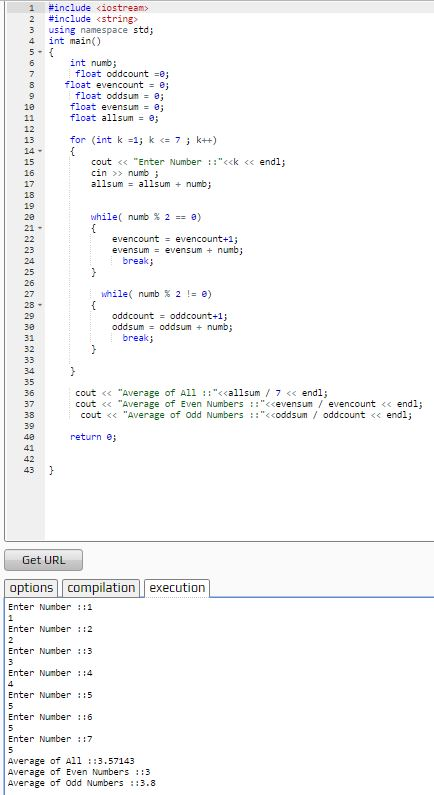# C++ Program to Average of 7 Numbers, Average of Odd Numbers and Average of Even numbers

```#include <iostream>
#include <string>
using namespace std;
int main()
{
int numb;
float oddcount =0;
float evencount = 0;
float oddsum = 0;
float evensum = 0;
float allsum = 0;

for (int k =1; k <= 7 ; k++)
{
cout << "Enter Number ::"<<k << endl;
cin >> numb ;
allsum = allsum + numb;

while( numb % 2 == 0)
{
evencount = evencount+1;
evensum = evensum + numb;
break;
}

while( numb % 2 != 0)
{
oddcount = oddcount+1;
oddsum = oddsum + numb;
break;
}

}

cout << "Average of All ::"<<allsum / 7 << endl;
if (evencount != 0 )
cout << "Average of Even Numbers ::"<<evensum / evencount << endl;
else
cout << "No Even Numbers entered"<< endl;

if (oddcount != 0)
cout << "Average of Odd Numbers ::"<<oddsum / oddcount << endl;
else
cout << "No Odd Numbers entered"<< endl;

}```